# Abstract

An alternative method is proposed to characterize the radiation pattern of antennas from an impulse response, based on a moment expansion together with a frequency domain near-to-far-field transformation via a FDTD/CPML solver. The approach introduced in the present work is intended for printed antenna analysis in special cases when the geometries to be modeled require a high degree of discretization in the space-time domain, which directly affects the frequency resolution when using FFT to perform the conventional NF-FF techniques. Results are compared with those obtained by a reference frequency-domain NF-FF technique and the applicability of the proposed method is demonstrated.

Finite-difference time-domain method; time-domain moment expansion; near-to-far-field transformation

Characterization of the radiation pattern of antennas via FDTD and time-domain moment expansion

Glaucio L. RamosI; Cássio G. RegoII

IFederal University of São João del-Rei, Department of Telecommunications and Mechatronics Engineering, Ouro Branco, MG, glaucio@ufsj.edu.br

IIFederal University of Minas Gerais, Department of Electronic Engineering, Belo Horizonte, MG, cassio@cpdee.ufmg.br

ABSTRACT

An alternative method is proposed to characterize the radiation pattern of antennas from an impulse response, based on a moment expansion together with a frequency domain near-to-far-field transformation via a FDTD/CPML solver. The approach introduced in the present work is intended for printed antenna analysis in special cases when the geometries to be modeled require a high degree of discretization in the space-time domain, which directly affects the frequency resolution when using FFT to perform the conventional NF-FF techniques. Results are compared with those obtained by a reference frequency-domain NF-FF technique and the applicability of the proposed method is demonstrated.

Index Terms: Finite-difference time-domain method, time-domain moment expansion, near-to-far-field transformation

I. INTRODUCTION

The finite-difference time-domain (FDTD) method in addition to absorbing boundary conditions and perfect matched layers (PML) has been successfully used in the analysis of radiation and scattering problems . FDTD is very efficient when making near field calculations, while for the far field it is necessary to use an additional method to perform a near-to-far-field (NF-FF) transformation.

The first technique used to perform the NF-FF transformation could only be applied to a single frequency analysis , . In order to obtain a broader frequency bandwidth response, a broadband excitation followed by a discrete Fourier transform was performed , . Recently, methods involving a recursive addition of tangential field contribution over a virtual equivalent surface have been used to perform the same task -. There are basically two different methods to perform a NF-FF transformation . In the first approach the time-domain near electromagnetic fields over a virtual surface were obtained, followed by the calculation of the equivalent currents, and the calculation of the time-domain electromagnetic far fields with a time-domain NF-FF transformation. This procedure is adopted when broadband results are required. In the second method FFT is applied to the FDTD electromagnetic near fields. Therefore the equivalent currents are calculated in frequency-domain and the result is used to compute the far fields via a frequency-domain NF-FF transformation. This procedure is suited for the analysis of narrowband antennas. Conventional time-domain NF-FF transformation techniques , , where time-domain far fields can be directly obtained, are based on the retarded potential method and as consequence, a new integration over the near-field is necessary for each observation point , . Therefore, when broadband results are required at a large number of observation angles, as in the case with radiation patterns, these techniques require a larger amount of computational resources .

Here an alternative method is proposed, based on frequency domain NF-FF transformation together with moment expansion of a Gaussian pulse to characterize the radiation patterns of wideband antennas. The method is intended for printed antenna analysis, in special cases when the geometries to be modeled on FDTD Yee cells require a high degree of discretization in the space-time domain, which directly affects the frequency resolution when using FFT to perform the frequency-domain NF-FF transformation. The proposed moment expansion allows us to estimate, for a single frequency, the equivalent near fields on a Huygens surface before being applied to the near-to-far-field transformation. Therefore, the frequency-domain NF-FF allow us to calculate the radiation pattern of a broadband antenna for a large frequency band. Also it is an alternative technique to obtain the frequency-domain radiation pattern for broadband antennas without the need to perform costly time-domain numerical computation , .

This paper is organized as follows: Section II gives a brief description of the frequency-domain near-to-far-field transformation. In Section III, the radiation patterns obtained from two UWB antennas are presented and compared to results from the time-domain moment expansion technique.

II. FORMULATION OF THE FREQUENCY DOMAIN NEAR-TO-FAR-FIELD TRANSFORMATION

To perform the frequency-domain near-to-far-field transformation, the field components on a Huygens surface are replaced by equivalent electric and magnetic dipoles , allowing the calculation of the equivalent surface currents moments by using FDTD near fields. Once the surface current moments are determined, the vector potential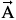andare used to calculate the radiation far fields . Here we suggest an alternative approach to perform the near-to-far-field transformation by the use of a moment expansion process, which uses a fourth order approximation to estimate the near fields from its impulse response .

When performing antenna analysis via a FDTD it is necessary to minimize numerical dispersion. The number of chosen points per wavelength for the cell size is dependent on many factors, but a good rule of thumb is 10 points per wavelength . In order to avoid numerical instability the time step must also be selected as Δt < Δ / c, where Δx = Δy = Δz = Δ and c is the speed of light at vacuum . The use of the previous space discretization leads to a FDTD time step of Δt < λ / (10c), which has a direct impact on frequency resolution when using FFT to perform the conversion from time to frequency domain. As the maximum frequency of the sampled signal is fmax = 1 / 2Δt = 5c/ λ, and using N time samples, the frequency resolution after the FFT process is fmax / N. When using FDTD to analyze some special cases of printed antenna geometries it is necessary to perform a degree of space discretization significantly higher than the minimum necessary to minimize numerical dispersion, in order to make a proper antenna modeling. This leads to a decrease in the FFT frequency resolution and thus has an impact on the results obtained from the frequency domain radiation pattern of these kinds of antennas and suggests the use of the moment expansion technique in order to improve the accuracy of the results.

It was also shown that as a Gaussian pulse is band-limited, the computation of the frequency-domain near fields in the FDTD solver is less accurate outside a frequency band depending on the chosen computational parameters . As a consequence, the moment expansion technique with fourth-order truncation has been shown to improve accuracy and computational efficiency when using Gaussian pulse excitation as compared with the Fourier transform, especially when the upper frequency of the impulse response is greater than its upper (10% amplitude) frequency -. The parameters used for the impulse response retrieval via FDTD and moment expansion deconvolution technique may be> 1.5 fm, where=, n may be in the range {8-10}, Δ is the largest FDTD cell size and fm is the highest frequency to be computed. The Gaussian-pulse parameters may also be such that its 10% amplitude upper frequency fG may be in the range of [1.5. fm,] .

III. MOMENT EXPANSION OF TIME FUNCTIONS AND IMPULSE RESPONSE

The impulse response of an antenna can be obtained by an inverse Fourier transform in a deconvolution process. It can be also be obtained directly from an arbitrary time response of the antenna under analysis, by a moment expansion of a signal in the time domain . Considering a circuit excited at a port i with a signal vi(t) and the associated response, vk(t), at a port k, the followed relation is valid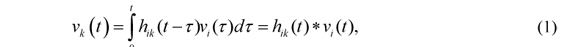where hik(t) is the impulse response between k and i ports written aswith ℑ-1 being the inverse Fourier transform, Vi(ω) = ℑ [vi(t)], and Vk (ω) = ℑ[vk(t)]. The impulse response hik(t) can be obtained by FDTD using a Fourier process for the samples vi(nΔt) and vk(nΔt), or the integral equation as in .

A deconvolution with less computational effort can be obtained using the expansion as in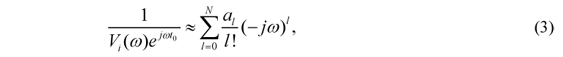where t0 and the coefficients a1 are obtained by the moments µm of the time signal vi(t):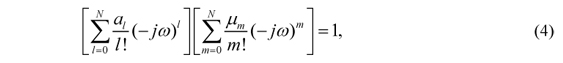withandUsing the moment expansion, the impulse response as in (2) can be written as:or, for vi(t) with non-zero average: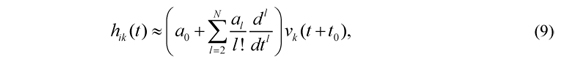or, for the zero average case:In this work the input signal for the antenna under analysis was the gaussian pulse in the form of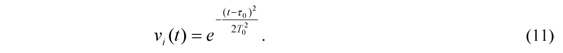Using the FDTD time increment t = nΔt, n = 0, 1, ..., and for a Gaussian pulse excitation in the form of (11) we can use a fourth order approximation for the input i and output port k leading to an impulse response between these ports hik aswhere the parameters a0, a2, a4 and n0 are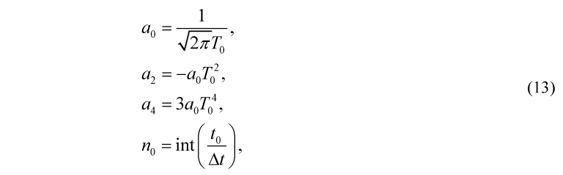and the shift parameter t0= jV(1) (0) / V (0), with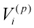(0) =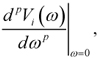is chosen as .

The moment expansion technique was applied to the antenna problem analysis in such a manner that vi(t) is the antenna pulse excitation and vk(t) is each near field component on a virtual surface surrounding the antenna. The impulse response is then estimated for each near field component and after the convolution process each near field component is properly replaced as an input to the NF-FF transformation.

IV. NUMERICAL RESULTS

The moment expansion and frequency-domain near-to-far-field techniques were applied to two UWB patch antennas in order to obtain their radiation pattern , .

The patch antenna dimensions for the geometry of Figure 2 are Lsub = 35 mm, Wsub = 30 mm, Lf = 12.5 mm, Wf = 3.2 mm, Lp = 14.5 mm, Wp = 15 mm, Wc = 1 mm, Lst1 = 1 mm, Wst1 = 1.5 mm, Lst2 = 1.5 mm, Wst2 = 1.5 mm, Lsl1 = 5 mm, Lsl2 = 7 mm and Wsl = 0.5 mm. This UWB antenna also has a 1.6 mm thick FR4 dielectric substrate, on a z-plane, with a relative permittivity of εr = 4.4. The antenna dimensions correspond to 140 × 120 × 3 FDTD cells, at x, y and z direction, respectively. To obtain the radiation pattern of the antenna under simulation, a FDTD/CPML reference code was obtained  and the full domain of the FDTD solver has 200 × 180 × 63 cells. It was also performed 2000 FDTD time iterations.

Considering the minimum FDTD discretization to minimize numerical dispersion, Δ = 3 mm, and instability,Δt < 5.7735 ps, the frequency resolution obtained at FFT procedure should be approximately 43.3 MHz. However, due to antenna dimensions the parameters used at simulation was Δ = 0.25 mm and Δt = 0.385 ps - a FDTD time step 15 times lower than the minimum. Thus the frequency resolution due to FFT was 650 MHz and the moment expansion was used to increase the accuracy of the results for the radiation pattern of the antenna under analysis. From the previously defined FDTD parameters, fm = 10 GHz is the highest frequency to be computed and= 1.2 × 1011 Hz, which follows the previously defined set of parameters that enables the use of impulse response retrieval via FDTD and ME deconvolution.

To include the moment expansion technique, the antenna was excited with a Gaussian pulse with T0 = 0.125 ps and τ0 = 0.796 ps, where its 10% amplitude upper frequency fG = 27.32 GHz is in the range [15 GHz, 57 GHz]. The proposed technique was performed according to the following steps: the near field was obtained by the FDTD solver. Once the impulse response of the system was known, a convolution with a sinusoidal carrier was performed and the near field was updated for the frequency under analysis. Finally, the frequency-domain near-to-far-field transformation was carried out yielding the radiation pattern. These steps are summarized in Fig. 1.

The radiation pattern of the UWB antenna under analysis was obtained by three different methods applied to the FDTD/CPML code: a sinusoidal carrier at 10 GHz as input signal (NF2FF CW, used as reference), a Gaussian pulse was used as input signal with the use of the moment expansion technique (NF2FF ME) and the same Gaussian pulse as input signal with the conventional DFT procedure (NF2FF Pulse). Figure 3 shows an excellent agreement between the proposed moment expansion technique and the NF-FF technique with a sinusoidal input signal and this better result can be explained by the convolution with a sinusoidal input source at the moment expansion process. The use of the proposed frequency-domain near-to-far-field together with the moment expansion is shown to be more numerically efficient than the conventional technique, when a Gaussian pulse is used as an input signal to characterize antenna radiation patterns in the cases when the modeling process of the antenna on FDTD Yee cells requires a high degree of space discretization.

Radiation patterns of the UWB antenna for the x-y, x-z and y-z planes at 4, 7 and 10 GHz were performed using the proposed moment expansion technique and compared with results obtained by a carrier input signal for each frequency under analysis, as well as with measuments previously done . Results are shown in Figs. 3-11. These results show excellent agreement between the proposed technique and previously obtained results . These results also show that the proposed moment expansion together with FDTD NF-FF solver, is able to efficiently calculate the radiation patterns in a wide range of frequencies for these special cases of printed antennas. The results obtained are very close to results from the reference single carrier excitation. Similar radiation patterns could be obtained by running the FDTD code for each frequency under analysis, but this method is not suitable for computation. The method proposed here shows that even if there is a need to perform one more step in characterizing the antenna radiation pattern, which is the convolution of the carrier frequency with the obtained moment expansion impulse response, the additional step increases the accuracy of numerical results without considerable increase to the computational effort. The differences between the results obtained by simulation with the proposed technique and measurements previously done are similar to the differences obtained when comparing the measurements with simulations obtained by a commercial software and was explained by the effect of the feeding cables, the feeding connector and the antenna fixation support .

The proposed technique was also applied to the antenna geometry of Figure 12. The antenna dimensions are L = 33 mm, Wg = 30 mm, L1 = 16.5 mm, W1 = 12 mm, L2 = 4 mm, W2 = 3 mm, Gw1 = 1.5 mm, Lg = 15 mm, Lsc = 2.25 mm, Ls1 = 4 mm, Ws1 = 2.5 mm, Ls = 4 mm, and Ws = 0.3 mm. This UWB antenna also has a 1.52 mm Taconic FR35 dielectric substrate, on a z-plane, with a relative permittivity of εr = 3.5. The antenna dimensions correspond to 60 × 66 × 3 FDTD cells, at x, y and z direction, respectively. To obtain the radiation pattern of the antenna under simulation, a FDTD/CPML reference code was obtained  and the full domain of the FDTD solver has 100 × 106 × 43 cells. It was also performed 2000 FDTD time iterations.

Radiation patterns of this UWB antenna for the x-y, x-z and y-z planes at 4, 7 and 10 GHz were obtained and results are shown in Figs. 13-21. These results show once more that the proposed moment expansion together with FDTD NF-FF solver, is able to efficiently calculate the radiation patterns in a wide range of frequencies for these special cases of printed antennas.

V. CONCLUSION

In this research a moment expansion of time signals was proposed to obtain the impulse response of some special cases of printed antennas and estimate the values of the near fields on a Huygens equivalent surface for each frequency where the radiation pattern is to be characterized. These near field estimated values were used together with a frequency-domain near-to-far-field transformation to characterize the radiation pattern of an UWB printed antenna at three different frequency bands, allowing the use of a frequency-domain NF-FF technique for wideband analysis.

The proposed technique is intended to printed antenna analysis for the special cases when the geometries to be modeled require a high degree of space discretization. Since the conventional NF-FF transformation are performed in frequency-domain, when using FDTD it is necessary FFT computation. For these specific antennas, the high discretization makes the frequency resolution coarser and the use of the moment expansion was used in order to increase the accuracy of the results of the radiation patterns. The proposed methodology was applied to two different UWB antennas and results were compared with conventional techniques. The results obtained were more numerically

precise, thus validating the use of the proposed moment expansion based technique in performing frequency-domain radiation patterns of antennas excited by a Gaussian pulse, even if there is an additional step in characterizing the antenna radiation pattern as there is no significant increase in computational effort.

When compared with conventional formulations, the proposed methodology has be shown to improve the accuracy of frequency domain radiation pattern analysis, for these special cases of printed antennas, as it permits a convolution with a sinusoidal input source, before the frequency domain NF-FF is applied. For time domain NF-FF, the moment expansion methodology has also been shown to improve accuracy and computational efficiency when compared with conventional Fourier transform, under the conditions mentioned before.

ACKNOWLEDGMENTS

This work was partially supported by CNPq, under Covenant 573939/2008-0 (INCT-CSF) and Project 300938/2008-0, and Fapemig, under Projeto do Pesquisador Mineiro.

Received 17 May 2013;

For review 17 May 2013;

Accepted 20 May 2014

•  A. Taflove and S. C. Hagness, Computation Electrodynamics: The Finite-Difference Time-Domain Method, Artech House, Boston, MA, 1995.
•  Roden and S. Gedney, "Convolution PML (CPML): an efficient FDTD implementation of the CFS-PML for arbitrary media," Microwave and Optical Technology Letters, vol. 27, no. 5, pp. 334-339, December 2000.
•  A. Taflove, "Application of the finite-difference time-domain method to sinusoidal steady state electromagnetic penetration problems," IEEE Transactions on Electromagnetic Compability, vol. 22, no. 3, pp. 191202, August 1980.
•  A. Elsherbeni and V. Demir, The Finite-Difference Time-Domain Method for Electromagnetics with MATLAB Simulations, Scitech Publishing, Raleigh, NC, 2009.
•  K. Yee, D. Inghmam and K. Shlager, "Time Domain extrapolation to the far field based on FDTD calculations," IEEE Transactions on Antennas and Propagation, vol. 39, no. 3, pp. 410413, March 1991.
•  R. Luebbers, K. Kunz, M. Schneider and F. Hunsberger, "A finite-difference time-domain near zone to far zone transformation," IEEE Transactions on Antennas and Propagation, vol. 39, no. 3, pp. 429433, March 1991.
•  M. Barth, R. McLeod and R. Ziolkowski, "A near and far field projection algorithm for finite-difference time-domain codes," Journal of Electromagnetic Waves and Applications, vol. 6, no. 16, pp. 518, March 1992.
•  D. Sullivan and J. L. Young, "Far-field time-domain calculation from aperture radiators using the FDTD method," IEEE Transactions on Antennas and Propagation, vol. 4, no. 3, pp. 464469, March 2001.
•  C. Oetting and L. Klinkenbusch, "Near-to-Far-Field Transformation by a Time-Domain Spherical-Multipole Analysis," IEEE Transactions on Antennas and Propagation, vol. 53, no. 6, pp. 20542063, June 2005.
•  J. Adam and L. Klinkenbusch, "Efficient evaluation of antenna fields by a time-domain multipole analysis," Advances in Radio Science, vol. 7, pp. 4348, May 2009.
•  C. A. Balanis, Antenna Theory: Analysis and Desing, Wiley-Interscience, 2nd ed., 2005.
•  G. Marroco and F. Bardati, "Time-domain macromodel of planar microwave devices by FDTD and moment expansion," IEEE Transactions on Microwave Theory and Techniques, vol. 49, pp. 13211328, July 2001.
•  G. Marroco and F. Bardati, "FDTD computation of microwave device impulse response," Electronics Letters, vol. 35, no. 3, pp. 223224, February 1999.
•  G. Talenti, "Recovering a function from a finite number of moments," Inverse Problems, vol. 3, pp. 501517, 1987.
•  T. Vuong, A. Ghiotto, Y. Duroc and S. Tedjini, "Design and characteristics of a small u-slotted planar antenna for IR-UWB," Microwave and Optical Technology Letters , vol. 49, no. 7, pp. 17271731, July 2007.
•  X. L. Bao, M. J. Ammann, "Printed UWB Antenna with Coupled Slotted Element for Notch-Frequency Function," International Journal of Antennas and Propagation, vol.2008, Article ID 713921, 8 pages, 2008.

# Publication Dates

• Publication in this collection
31 July 2014
• Date of issue
June 2014

# History

• Received
17 May 2013
• Accepted
20 May 2014
• Reviewed
17 May 2013
Sociedade Brasileira de Microondas e Optoeletrônica e Sociedade Brasileira de Eletromagnetismo Praça Mauá, n°1, 09580-900 São Caetano do Sul - S. Paulo/Brasil, Tel./Fax: (55 11) 4238 8988 - São Caetano do Sul - SP - Brazil
E-mail: editor_jmoe@sbmo.org.br